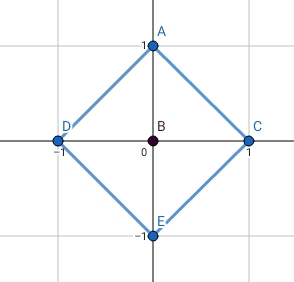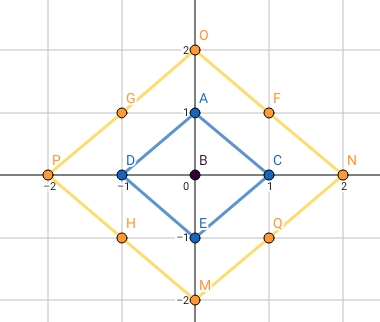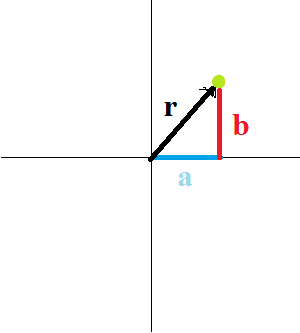# ZIO20001 - Editorial

Editorialist : Sudheera Y S

Prerequisites : Pattern finding

Problem statement :

Find the number of points whose manhattan distance is atmost S

Idea :

Let us slightly change our problem definition to - Answer for a number S is the number of points whose manhattan distance is exactly S

We are going to solve for S = 1…4 and we will find a pattern in it ( which is easy to prove also ) and then we will continue it and will compute the answerS = 0 : Answer = 1 - (0,0)

S = 1 : Answer = 4 - (1,0) , (0,1) , (-1,0) , (0,-1)

The points would look like this : ( Only Blue points )S = 2 : Anwer = 8

The points would be like this : ( Only Yellow points )S = 3 : Anwer = 12

The points with manhattan distance 3 would look like this : ( Only Red points )

S = 4 : Anwer = 16

The points with manhattan distance 4 would be like this : ( Only Green points )

Can we find some pattern here?

Let us consider all the points except the corners of the green square in the above diagram, we see that there are (S-1) points on each edge of the square and there are 4 edges so in total there are 4*(S-1) points and there are 4 corners which we are not considering, so the total number of points would be 4*(S-1) + 4 = 4*S

So the pattern is :

Answer for S = 4*S where S >= 1
And answer for S = 0 is 1

For finding the required answer for S, we need to add our answers from S = 0……S and that would be our final answer

S = 4 : Sum = 1 + 4 + 8 + 12 + 16 = 41

S = 10 : Here instead of adding all the numbers can we find a formula ??

Answer for S = 1 : 4*1

Answer for S = 2 : 4*2

Answer for S = 3 : 4*3

.

.

.

Answer for S = 10 : 4*10

Sum of all the answers = 41 + 42 + … + 4*10

= 4*(1+2+…+10)

= 4*(1011)/2 ( Sum of first n numbers is (n(n+1))/2 )

= 2*110

= 220

NOTE : We should remember to add 1 to our answer as S = 0 is not being considered in the formula

So the answer will be 221

S = 23

Applying the formula for S = 23 , we get ,

Sum of all answers = 4*(1+2+…+23)

= 4*(2324)/2 (Sum of first n numbers is (n(n+1))/2 )

= 2*552

= 1104

NOTE : We should remember to add 1 to our answer as S = 0 is not being considered in the formula

So the answer will be 1105

Hope you understood2 Likes

can i use beggar’s method in P&C to solve it?each manhattan dist = a+b
S=a+b
number of a,b satisfying = C(n+r-1,r-1)
= C(S+1,1)=S+1
sum over S+1 for s from 1 to S
do the same for other quadrants. However, my answer is wrong. I may’ve done a silly calc mistake.
But, is this approach correct?

Didnt understand what you are saying
Can you ps explain bit more clearly what you said
Thanks